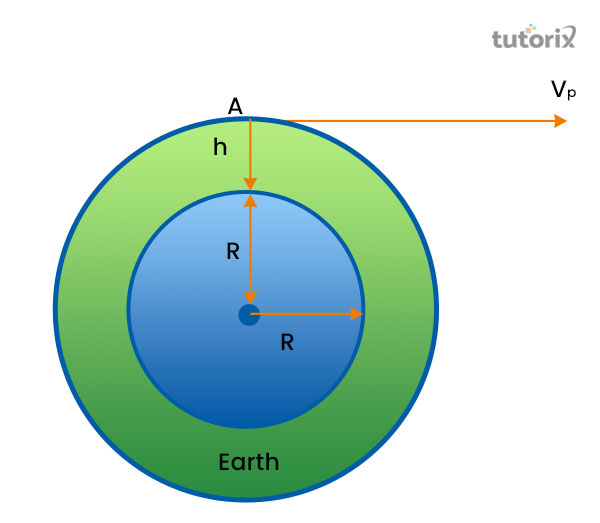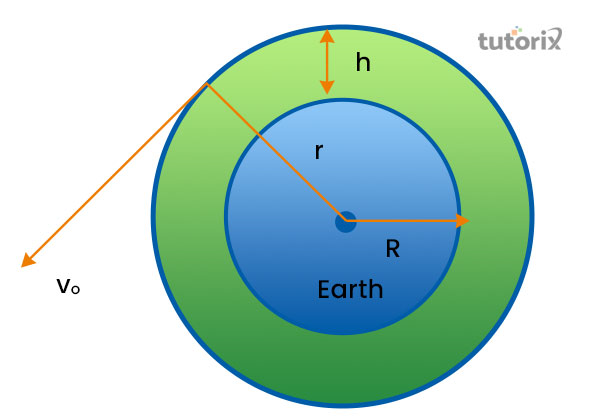# Relation between Escape Velocity and Orbital Velocity

## Introduction

The present tutorial intends to include the explanation regarding the concepts of physics such as escape velocity and orbital velocity along with explaining the relationship that lies between these two concepts. The tutorial will further include the definition of escape velocity and orbital velocity in order to build or exemplify the relation these two concept forms between themselves. Moreover, this tutorial will include an explanation of the formula of relation along with the differences between these concepts behold.

## What is Escape Velocity?Figure 1: Escape velocity

In simple diction, the minimum velocity can define the escape velocity that an orbiting object is required in order to escape from the respective orbit on which the object is rotating (Vlacic, 2019).

For being a free non-propelled object, in the terminology of celestial mechanist, the minimum speed required is referred to as the escape velocity of that particular object. The escape velocity of an object generally depends on the object's mass and size (Drolshagen et al. 2020).

The unit that is used in order to represent the escape velocity is meters per second. The application of this is seen in the exploration of space, as spacecraft need this escape velocity to go beyond the gravitational force of the earth.

## What is Orbital Velocity?Figure 2: Orbital velocity

The orbital velocity can be demonstrated as an aspect of a gravitationally bound system, where an object requires a specific amount of velocity that is considered the sufficient measure to remain in orbit (Sciencedirect, 2022).

To remain in motion, an object can be affected by inertia, the tendency to remain in motion, which can influence the path of orbiting that will be changed to a straight one. In such cases, to avoid the impact of inertia, the object needs a minimum velocity to remain in the path of the orbit.

The application of orbital velocity is seen in the shaping and orbit of natural or artificial starlight, on which these will remain orbiting the planets in space. The dependable of orbital velocity is the radius of the object, and the height of the object from the surface as well.

## Difference between Escape Velocity and Orbital Velocity

The concepts of escape velocity and the orbital velocity have several differences among themselves. The core difference that is identified is that the escape velocity is explained as the minimum velocity an object required to leave the gravitation force under which the object rotates. On the other hand, the orbital velocity refers to such amount of velocity that is GMR = vo2 by an object to remain in the orbit while maintaining its pace and the difference from the gravitational object (Geiger, 2019).

The formula of escape velocity is displayed as

ve = 2GMR

in contradiction, the formula of orbital velocity is ve = GMR + h. Based on the formulation of the mathematical formula of escape velocity, it also can be represented as 2gR. On the contrary, the representation of the orbital velocity can be done by the formula of GMR = vo2 as well.

## Escape Velocity and Orbital Velocity: Relation

The relation that is found between the orbital velocity and escape velocity is that the values of these two aspects are directly proportional to each other. The meaning of such statements is that the value of escape velocity will be increased if the value of the orbital velocity of the object will be increased. Similarly, if the orbital velocity of the object will be reduced, a reduction in the value of escape velocity will be seen as well. Based on these two conceptual ideas the formula that defines both the situations are

$$V_{o}= \sqrt{gR} \:and\: V_{e} = \sqrt{2gR}$$

## The Formula of the Relation between Escape Velocity and Orbital Velocity

The first equation $V_{o}= \sqrt{gR}$ represents the orbital velocity and the second formula $V_{e} = \sqrt{2gR}$ is responsible for the representation of escape velocity. In these two formulas, the letter g is referred to as the acceleration and R for the representation of radius, the main formula that can be formed is $V_{e} = \sqrt2 \sqrt{gR}$ (Haug, 2021). By substituting $V_{o}= \sqrt{gR}$ one can get $V_{e} = \sqrt2V_{o}$. Based on this, the value of orbital velocity can be found by the formula, $V_{o} = V_{e} /\sqrt2$.

## Conclusion

The tutorial has shed light on the definition of escape velocity, following this the tutorial further has included the definition of orbital velocity the relationship that lies between these two conceptualised aspects of astrophysics. The relation that lies between these two concepts has formulated a mathematical formula that states that the value of Escape velocity is equivalent to √2* Orbital velocity. This formula defines two relations that are formed, that states the increment or reduction of the value of escape velocity creates a similar impact on the valuation of the orbital velocity.

## FAQs

Q1. How escape velocity and orbital velocity are related?

The escape velocity of an object is calculated based on the orbital velocity as the gravitational force governs the increment or reduction of the orbital velocity. Taking under consideration of such conditions, the formed formula has stated that the value of escape velocity is equivalent to the product of orbital velocity and the square root of 2.

Q2. Which planet beholds the highest mark of escape velocity in the solar system?

In the solar system, the planet named Jupiter is the only planet that has the highest escape velocity which is 59.5 km per second.

Q3. What is the relation between orbital velocity and gravity?

The square root of universal gravitation shares a value that is equivalent to the orbital value of an object that is considered constant to the mass of the body, divided by the radius of the orbit.

Q4. On which factors does the escape velocity depend?

The escape velocity of an object generally depends on two attributes the object which is the size of the object along with its mass.

## References

### Journals

Drolshagen, E., Ott, T., Koschny, D., Drolshagen, G., Schmidt, A. K., & Poppe, B. (2020). Velocity distribution of larger meteoroids and small asteroids impacting Earth. Planetary and Space Science, 184, 104869. Retrieved from: https://arxiv.org/pdf/2011.07775

Geiger, J. (2019). Measurement Quantization Describes Galactic Rotational Velocities, Obviates Dark Matter Conjecture. Journal of High Energy Physics, Gravitation and Cosmology, 5(02), 473. Retrieved from: https://www.scirp.org/html/13-2180374_91777.htm

Haug, E. G. (2021). New full relativistic escape velocity and new Hubble related equation for the universe. Physics Essays, 34(4), 502-514. Retrieved from: https://hal.archives-ouvertes.fr/hal-03240114/document

Vlacic, N. (2019). Escape Velocity. Undergraduate Journal of Mathematical Modeling: One+ Two, 3(1), 24. Retrieved from: https://scholar.archive.org

### Websites

Sciencedirect.com, (2022). Orbital Velocity - an overview Retrieved from: https://www.sciencedirect.com/topics/physics-and-astronomy/orbital-velocity [Retrieved on 11th June 2022]

Updated on: 13-Oct-2022

577 Views